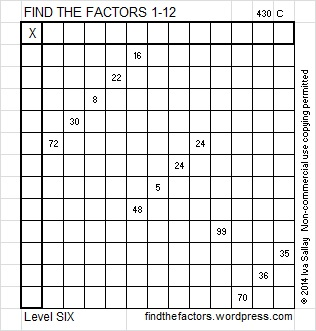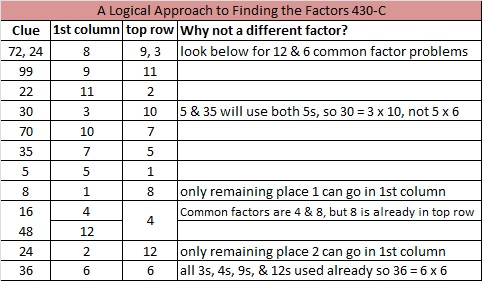# 191 and Level 6

• 191 is a prime number.
• Prime factorization: 191 is prime.
• The exponent of prime number 191 is 1. Adding 1 to that exponent we get (1 + 1) = 2. Therefore 191 has exactly 2 factors.
• Factors of 191: 1, 191
• Factor pairs: 191 = 1 x 191
• 191 has no square factors that allow its square root to be simplified. √191 ≈ 13.82027How do we know that 191 is a prime number? If 191 were not a prime number, then it would be divisible by at least one prime number less than or equal to √191 ≈ 13.8. Since 191 cannot be divided evenly by 2, 3, 5, 7, 11, or 13, we know that 191 is a prime number.

There are 22 prime numbers between 100 and 200:

• The first four of those prime numbers are 101, 103, 107, and 109.
• The last four of those prime numbers are 191, 193, 197, and 199.Excel file of puzzles and previous week’s factor solutions: 12 Factors 2014-07-28This site uses Akismet to reduce spam. Learn how your comment data is processed.